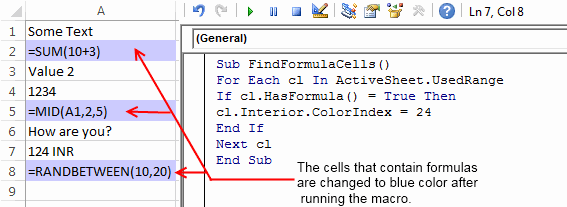# How to Find Cells containing Formulas in Excel

A few days back I had a huge spreadsheet in which I had to find out the cells containing formulas. Initially, I was totally clueless about how this task can be done.

But later after doing some Google searches, I got a few ideas on how I can effortlessly identify the formula cells in excel.

And today to share my experiences with you guys, In this post I will throw some light on a few methods that can help you to find out formula cells in your spreadsheets.

So here we go:

## Method 1: Using ‘Go To Special’ Option:

In Excel ‘Go To Special’ is a very handy option when it comes to finding the cells with formulas. ‘Go to Special’ option has a radio button “Formulas” and selecting this radio button enables it to select all the cells containing formulas.

Later you can change the formatting or background color of the selected cells to make them stand out from the rest. Below is the step by step instructions for accomplishing this:

1. With your excel sheet opened navigate to the ‘Home’ tab > ‘Find & Select’ > ‘Go To Special’. Alternatively, you can also press ‘F5’ and then ‘Alt + S’ to open the ‘Go to Special’ dialog.2. Next, in the ‘Go to Special’ window select the ‘Formulas’ radio button. After checking this radio button you will notice that few checkboxes (like Numbers, Errors, Logical, and Text) are enabled, these checkboxes signify the return type of the formulas.

So, if you select the ‘Formulas’ radio button and only check the ‘Numbers’ checkbox then it will just search the Formulas whose return type is a number. Here in our example, we will keep all of these return types checked.

3. After this click the ‘Ok’ button and all the cells that contain formulas get selected.

4. Next, without clicking anywhere on your spreadsheet change the background color of all the selected cells.

5. Now your formula cells can be easily identified.

## Method 2: Using a built-in Excel formula

If you have worked with excel formulas then probably you may be knowing that excel has a formula that can find whether a cell contains a formula or not. The formula that I am talking about is:

`=ISFORMULA(reference)`

Here ‘reference’ signifies the cell position which you wish to check for the presence of a formula.

For example: If you wish to check the cell ‘A2’ for the existence of a formula then you can use this function as

`=ISFORMULA(A2)`

This function results in a Boolean output i.e. True or False. True signifies that the cell contains formulas while False tells that cell doesn’t contain any formulas.

## Method 3: Using a Macro for identifying the cells that contain formulas:

I have created a VBA Macro that can find and color any cells that contain the formula in the total used range of the Active sheet. To use this macro simply follow the below procedure:

1. Open your spreadsheet and hit the ‘Alt + F11’ keys to open the VBA editor.2. Next, navigate to ‘Insert>Module‘ and then paste the below macro in the editor.

`Sub FindFormulaCells()For Each cl In ActiveSheet.UsedRangeIf cl.HasFormula() = True Thencl.Interior.ColorIndex = 24End IfNext clEnd Sub`

3. For running this formula press the “F5” key.

4. This Macro will change the background colour of all the formula containing cells and thus makes it easier to identify them easily.About Ankit Kaul

Ankit is the founder of Excel Trick. He is tech Geek who loves to sit in front of his square headed girlfriend (his PC) all day long. :D. Ankit has a strong passion for learning Microsoft Excel. His only aim is to turn you guys into 'Excel Geeks'.